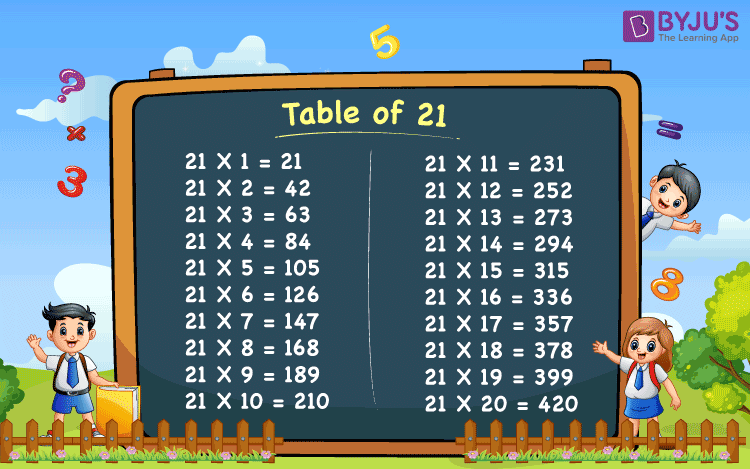Checkout JEE MAINS 2022 Question Paper Analysis : Checkout JEE MAINS 2022 Question Paper Analysis :

# Table of 21

Table of 21 is the 21 times table that includes the multiples of 21. The multiples of 21 can be produced by multiplying 21 with each of the natural numbers starting from 1.

In schools, from the very primary classes, we start learning about Maths tables. These maths tables basically give the values of the repeated addition of a number. In the same way, the maths table of 21, gives the repeated addition of the number 21.

For example, 3 rows of 21 boys each gives the sum of 21 + 21 + 21 = 63. Therefore, the total number of boys is 63. Or we can also write it as, 3 x 21 = 63, where 3 is the number of rows and 21 is the number of boys standing in each row.

## Table of 21 Chart## Multiplication Table of 21

The multiplication table of 21 is very useful for higher classes, say from 9th to 12th, as the maths concepts for these classes have a little higher level than that of primary classes. Students, who have memorized the 21 times table can also easily memorize the later tables such as the table of 22, 23, 24, 25 so on till 30. Also, students or candidates, who are preparing to attempt competitive exams like Bank Exams, CAT, MAT and other competitive exams which includes maths aptitude questions, can memorize the tables from 21 to 30 for quick calculations.

## 21 Times Table

Let us draw the table of 21 from numbers 1 to 20 times.

 21 x 1 = 21 21 x 2 = 42 21 x 3 = 63 21 x 4 = 84 21 x 5 = 105 21 x 6 = 126 21 x 7 = 147 21 x 8 = 168 21 x 9 = 189 21 x 10 = 210 21 x 11 = 231 21 x 12 = 252 21 x 13 = 273 21 x 14 = 294 21 x 15 = 315 21 x 16 = 336 21 x 17 = 357 21 x 18 = 378 21 x 19 = 399 21 x 20 = 420

In this table you can see, 21 is the multiplicand and the numbers from 1 to 20 are multipliers. So, in the table of 21, the number 21 is multiplied to the other numbers to get the results.

## Tips & Tricks to Memorise Table of 21

Memorising a table of 21 is an easy task.

• Unit place digit of the multiple of 21 is the same as the whole number that is multiplied to it. For example, 21 x 3 = 63.
• The pattern of the table of 21 is such that at the unit place, the digits are consecutive (when we proceed with its multiples). 21, 42, 63, 84, 105, and so on

## Solved Examples

Q.1: What is the value of 21 times 3 added to 21 times 5?

Solution: 21 times 3 = 21 x 3 = 63

21 times 5 = 21 x 5 = 105

Sum = 63 + 105 = 168

Q.2: Evaluate: (21 x 10) – (21 x 6)

Solution: 21 x 10 – 21 x 6

= 210 – 126

= 84

## Practice Questions (Worksheet)

1. If Ram brings 21 candies each for his 4 friends, how many total candies does he have?
2. What are the 21 times 8?
3. What is the value of 21 times 9 subtracted by 21 times 22?

Download BYJU’S – The learning app and learn new techniques to learn Maths.

## Frequently Asked Questions on Table of 21

### What is the table of 21?

The table of 21 is the multiplication table that includes the multiples of 21, when multiplied by the whole numbers.

### What is 21 times table?

21 times table is the table of multiples of 21, which represent the repeated addition of 21.

### What is 21 times 4?

21 times 4 is 84.
21 x 4 = 84.

### What is 21 times 0?

21 times 0 is 0, only.
21 x 0 = 0.

### What is three times of 21?

Three times of 21 is equal to 63.
21 x 3 = 63
Test your knowledge on Table Of 21